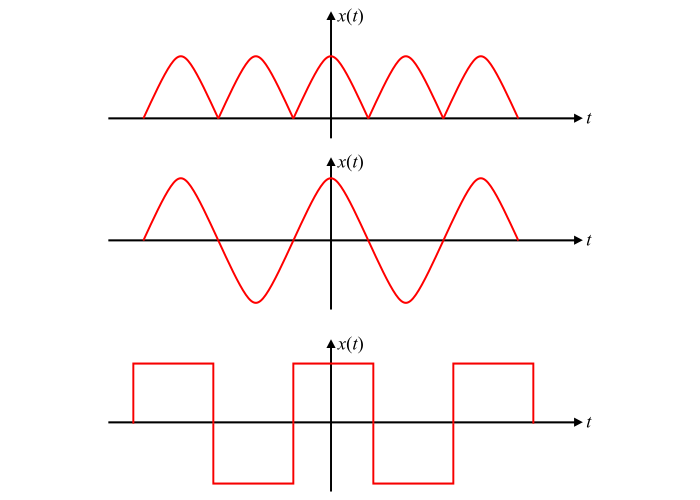# Signals and Systems â€“ What is Even Symmetry?

## Importance of Wave Symmetry

If a periodic signal đť‘Ą(đť‘ˇ) has some type of symmetry, then some of the trigonometric Fourier series coefficients may become zero and hence the calculation of the coefficients becomes simple.

## Even or Mirror Symmetry

When a periodic function is symmetrical about the vertical axis, it is said to have even symmetry or mirror symmetry. The even symmetry is also called the reflection symmetry. Mathematically, a periodic function x(t) is said to have even symmetry, if

$$\mathrm{đť‘Ą(đť‘ˇ) = đť‘Ą(−đť‘ˇ)\:\:\:\:\:\: ...(1)}$$

Some examples of functions having even symmetry are shown in the figure. The even functions are always symmetrical about the vertical axis.## Explanation

As we know that any periodic signal đť‘Ą(đť‘ˇ) can be split into even and odd components, i.e.,

$$\mathrm{đť‘Ą(đť‘ˇ) = đť‘Ą_{đť‘’}(đť‘ˇ) + đť‘Ą_{đť‘ś}(đť‘ˇ) … (2)}$$

If the function đť‘Ą(đť‘ˇ) is an even function, then,

$$\mathrm{đť‘Ą_{đť‘ś}(đť‘ˇ) = 0}$$

$$\mathrm{\therefore\:đť‘Ą(đť‘ˇ) = đť‘Ą_{đť‘’}(đť‘ˇ)\:\:\:\:\:\: … (3)}$$

Therefore, the trigonometric Fourier series coefficients are given by,

$$\mathrm{đť‘Ž_{0}\: =\:\frac{1}{đť‘‡}\int_{-\frac{đť‘‡}{2}}^{\frac{đť‘‡}{2}}đť‘Ą(đť‘ˇ)\: dt\:=\: \frac{1}{đť‘‡}\:\int_{-\frac{đť‘‡}{2}}^{\frac{đť‘‡}{2}}đť‘Ą_{e}(đť‘ˇ)\: dt}$$

$$\mathrm{⇒đť‘Ž_{0}\: =\:\frac{1}{đť‘‡}(2\int_{0}^{\frac{đť‘‡}{2}}đť‘Ą_{e}(đť‘ˇ) \: dt)\:=\:\frac{2}{đť‘‡}\int_{0}^{\frac{đť‘‡}{2}}đť‘Ą(đť‘ˇ) \: dt}$$

$$\mathrm{\therefore đť‘Ž_{0}\: =\:\frac{2}{đť‘‡}\int_{0}^{\frac{đť‘‡}{2}}đť‘Ą(đť‘ˇ) \: dt\:\:\:.....(4)}$$

The coefficient đť‘Žđť‘› is given by

$$\mathrm{đť‘Ž_{đť‘›}\: =\:\frac{2}{đť‘‡}\int_{-\frac{đť‘‡}{2}}^{\frac{đť‘‡}{2}}đť‘Ą(đť‘ˇ) \cos\:n\omega_{0}t\: dt\:=\:\frac{2}{đť‘‡}\int_{-\frac{đť‘‡}{2}}^{\frac{đť‘‡}{2}}đť‘Ą_{e}(đť‘ˇ) \cos\:n\omega_{0}t\: dt}$$

$$\mathrm{đť‘Ž_{đť‘›}\: =\:\frac{2}{đť‘‡}(2\int_{0}^{\frac{đť‘‡}{2}}đť‘Ą_{e}(đť‘ˇ) \cos\:n\omega_{0}t\: dt)}$$

$$\mathrm{\therefore đť‘Ž_{đť‘›}\: =\:\frac{4}{đť‘‡}\int_{0}^{\frac{đť‘‡}{2}}đť‘Ą(đť‘ˇ) \cos\:n\omega_{0}t\: dt\:\:\:.....(5)}$$

The coefficient đť‘Źđť‘› is given by,

$$\mathrm{đť‘Ź_{đť‘›} =\:\frac{2}{đť‘‡}\int_{-\frac{đť‘‡}{2}}^{\frac{đť‘‡}{2}}đť‘Ą_{e}(đť‘ˇ) \sin\:n\omega_{0}t\: dt}$$

Since the function (đť‘Ąđť‘’(đť‘ˇ) sin đť‘›đťś”0đť‘ˇ) is an odd function.

$$\mathrm{\therefore đť‘Ź_{đť‘›}\:=\:\frac{2}{đť‘‡}\int_{-\frac{đť‘‡}{2}}^{\frac{đť‘‡}{2}}đť‘Ą_{e}(đť‘ˇ) \sin\:n\omega_{0}t\: dt\:= 0\:\:\:.....(6)}$$

Hence, the Fourier series expansion of an even periodic function contains only a constant and cosine terms. Also, when the even or mirror symmetry exists in a function, then the trigonometric Fourier series coefficients for the function are given by equations (4), (5) and (6).

It is clear from the above discussion that when even symmetry exists in the function, then the trigonometric Fourier coefficient đť‘Źđť‘› becomes zero and thus the calculation becomes simple.

## Properties of Even Functions

• The sum of two or more even functions is always even.
• The product of two even functions is always even.
• When a constant is added to an even function, the even nature of the function still persists.